Zhang, L., Jia, Y., He, H., Yin, J., Chen, R., Zhang, C., Shen, W., and Wang, X. (2018). "Multiple factor analysis on preparation of cellulose Nanofiber by ball milling from softwood pulp," BioRes. 13(2), 2397-2410.

#### Abstract

This work aimed to investigate the effects of various single factors and their interactions on the preparation of cellulose nanofiber (CNF) using ball milling. These factors included the milling ball size, milling time, mass ratio of milling ball to cellulose pulp, and alkali concentration, as well as their complicated interactions. The Taguchi method was applied to the experimental design to systematically study the influences of these factors on the yield of CNF. A method for evaluating the yield of CNF was developed. Both of the simple analysis (SA) and analysis of variance (ANOVA) of the experimental results indicated that the most significant factor influencing the yield of CNF was the mass ratio of milling ball to cellulose pulp. The interactions between this factor and other single factors, e.g., milling time, also showed significant influence on the yield of CNF. Although the influence of milling ball size was negligible, its interaction with mass ratio of milling ball to cellulose pulp must be considered during production. Based on these findings, a mini scaled-up practice was proposed to demonstrate the possibility of the application of ball milling on the mass production of CNF.

Multiple Factor Analysis on Preparation of Cellulose Nanofiber by Ball Milling from Softwood Pulp

Liyuan Zhang,a,b,§,* Yumei Jia,c,§ Hui He,b Juliang Yin,d,* Ruoyang Chen,Caiqian Zhang,c Wei Shen,b and Xungai Wang a

This work aimed to investigate the effects of various single factors and their interactions on the preparation of cellulose nanofiber (CNF) using ball milling. These factors included the milling ball size, milling time, mass ratio of milling ball to cellulose pulp, and alkali concentration, as well as their complicated interactions. The Taguchi method was applied to the experimental design to systematically study the influences of these factors on the yield of CNF. A method for evaluating the yield of CNF was developed. Both of the simple analysis (SA) and analysis of variance (ANOVA) of the experimental results indicated that the most significant factor influencing the yield of CNF was the mass ratio of milling ball to cellulose pulp. The interactions between this factor and other single factors, e.g., milling time, also showed significant influence on the yield of CNF. Although the influence of milling ball size was negligible, its interaction with mass ratio of milling ball to cellulose pulp must be considered during production. Based on these findings, a mini scaled-up practice was proposed to demonstrate the possibility of the application of ball milling on the mass production of CNF.

Keywords: Ball milling; Cellulose nanofiber (CNF); Multiple factor analysis (MFA); Taguchi method; Analysis of variance (ANOVA)

Contact information: a: Institute for Frontier Materials, Deakin University, Geelong, Victoria 3216, Australia; b: Department of Chemical Engineering, Monash University, Melbourne, Victoria 3800, Australia; c: Shaoxing University, Shaoxing, Zhejiang 312000, China; d: Department of Statistics, School of Economics and Statistics, Guangzhou University, Guangzhou 510006, China;

§ Both authors contribute equally as co-first authors

* Corresponding authors: liyuan.zhang@monash.edu; yin_juliang@hotmail.com

INTRODUCTION

Cellulose nanofiber (CNF) has a high aspect ratio with its width and length measured on nanometer and micrometer scales, respectively (Salas et al. 2014). It has exceptional mechanical and surface properties, e.g., low density, high strength and stiffness, and high surface area-to-volume ratio, as well as potential compatibility with other materials (Salas et al. 2014; Abitbol et al. 2016). As a result of these specific advantages, CNF has been found effective in numerous applications including tissue engineering (Bodin et al. 2007), thermal insulation materials (Nogi et al. 2013; Wicklein et al. 2015), surface enhanced Raman scattering (SERS) substrates (Zhang et al. 2015a; Chen et al. 2017), rheological modifiers (Boluk et al. 2011), filtration media (Wertz and Schneiders 2009), and wound dressing (Brown et al. 2006; Czaja et al. 2006). To manufacture CNF and realize its potential applications, various methods have been proposed, e.g., electrospinning (Kim et al. 2006), biosynthesis (Brown 1886), and mechanical/ chemical isolation (Chen et al. 2011). However, each of these methods has its own critical limitations that prevent the above-mentioned applications and mass production of CNF (Siró and Plackett 2010; Abdul et al. 2012; Lavoine et al. 2012). Therefore, a preceding work conducted research on producing CNF using ball milling, which is a facile, simple, and efficient technique (Zhang et al. 2010, 2012).

Another previous study revealed the major influencing factors on the quality and yield of CNF: milling ball size, milling time, mass ratio of milling ball to cellulose pulp, and alkaline pretreatment (Zhang et al. 2015b). The previous study mainly focused on the influences of the above four major factors on the quality of CNF. Ball milling is a well-established technique for producing particles of a low aspect ratio. The success in using this simple and economic technique for CNF production was unexpected, and the reason behind the observed effects was intriguing. The previous study indicated that the above single factors on the milling process determined the yield of CNF, and their interactions also influenced the manufacturing process. To explore these influences, it is necessary to examine all of the major influencing factors collectively to analyze the combined effects caused by their interactions of multiple factors on the CNF yield.

The Taguchi method was applied in the design of experiments (DoE), and the multiple factor analysis (MFA) was used to tailor the multiple sets of variables collected and analyze the interactions between these sets of variables (Abdi et al. 2013). The Taguchi method uses an orthogonal array to statistically design the experiments by reducing the experimental runs based on a mathematical model in evaluating the relationship among multiple influencing factors (Yang and Tarng 1998; Zirehpour et al. 2014). Meanwhile, an analysis of variance (ANOVA), which is a set of statistical methods, is normally employed to analyze the experimental data and to evaluate the significance of different factors as well as their interactions (Rama and Padmanabhan 2012; Kuznetsova et al. 2015).

In this study, the orthogonal arrays for the preparation of CNF by ball milling were designed by the Taguchi method, and the data were collected after obtaining scanning electron microscopy (SEM) images of CNF samples. The evaluating method in terms of responses was subsequently established for describing the CNF yield produced using this technique. All of the responses were statistically analyzed using the SPSS software package. The discussions on the simple analysis (SA) and ANOVA were included to find out the most significant influencing factor and the combined effects caused by the interaction between the factors on the yield of CNF. A mini scale-up production was then performed to simulate the mass production of CNF.

EXPERIMENTAL

Materials

DoE by the Taguchi method

The detailed information of CNF preparation from softwood pulp by ball milling was provided in a previous study (Zhang et al. 2015b). In this work, the mass of 5 wt.% cellulose suspension was set as 5 g for ball milling. The mass ratio of milling ball to cellulose was, therefore, controlled by adjusting the mass of milling ball. Based on the Single Factor Analysis (SFA) on the CNF yield by ball milling, four major influencing factors with possible interactions and their reasonable values were obtained, as listed in Table 1. Therefore, three-level orthogonal array L27 (3 × 4) was selected. The DoE of the MFA on ball milling are listed in Table 2. Accordingly, the experimental array of ball milling was conducted. Details of all experimental runs are listed in Table 3.

Table 1. Influencing Factors of Ball Milling and Their Reasonable Values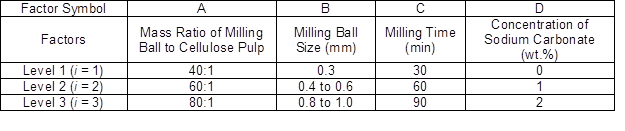Table 2. Experimental Layout Using Three-level Orthogonal Array L27 (3 × 4)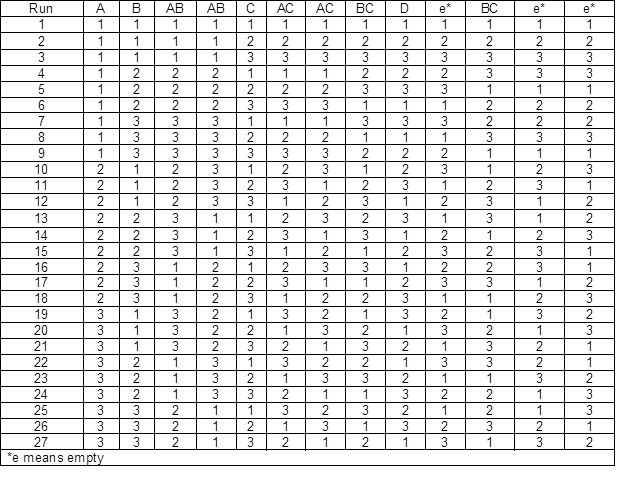Methods

Collection of responses

The SEM (Zeiss, Supra 55VP, Oberkochen, Germany) images with different magnifications (2,000×, 10,000×, 20,000×, and 50,000×) of all samples in a diameter of approximately 3 mm were analyzed. All of the cellulose fibers and particles in the SEM images were measured and counted. The term Fm was defined as the frequency percentage of CNFs with diameters less than 100 nm in all of the observed fibers and particles. In this study, Fm was calculated and regarded as the response for this orthogonal test, which also indicated the yield of CNFs for each experimental run.

Table 3. Experimental Matrix of Each Run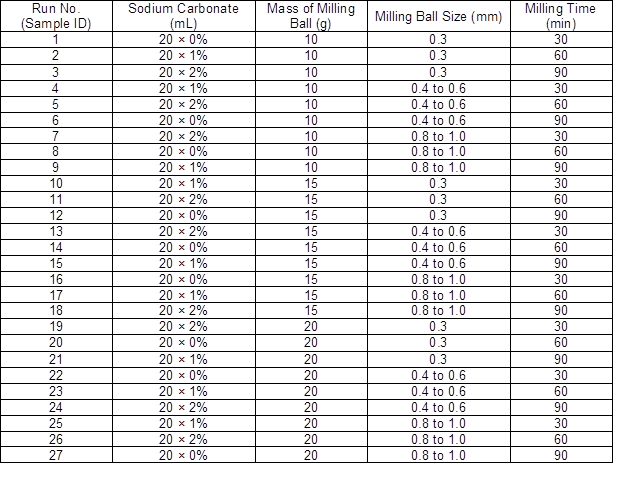All of the responses were filled in the response column of the data collection and calculation sheets and analyzed using the SPSS software package (IBM, version 19, Durham, USA). For this test, the criteria α was selected as 0.10, which meant that the confidence interval was 90%.

Mini scale-up production

To make CNF paper, the preparation of CNF needs to be scaled-up. In this work, 45 g of a cellulose pulp suspension was made using Northern bleached softwood kraft NIST reference material 8495 (pulp mill, Grande Prairie, Alberta, Canada) with a solid content of 1.0%. It was then mixed with 30 g of yttrium-doped-zirconia (YDZ; Klausen, Blaxland, Australia) milling ball with a diameter of 0.3 mm. The milling time was 1.5 h without any other chemicals. After mechanical treatment, the suspension was diluted by 100 fold using Millipore water (18.2 MΩ·cm; Merck, Ziefen, Switzerland). Subsequently, the fiber suspension was successively passed through the mesh screen with 250 μm openings to remove any large fibers. The filtrate was then centrifuged to concentrate the CNF suspension. The typical solid content of the CNF suspension after centrifuging was between 1.4% and 1.5% for the subsequent characterization and analysis of CNF.

RESULTS AND DISCUSSION

Evaluation of Tested Samples for Orthogonal Tests

All runs of ball milling were performed following the experimental matrix listed in Table 3. One typical SEM image of the obtained products, i.e., sample No. 23, was selected to demonstrate the calculation of the response factor (Fm), as shown in Fig. 1. The image clearly shows that the CNFs (yellow arrows), large fibers (blue arrows), and cellulose particles (red circles) were produced using ball milling at the same time; these three major products can be distinguished easily from the SEM images. There are many variances that therefore should be compared, e.g., the presence of CNFs, large fibers, and cellulose particles, as well as their respective amounts. However, if all of these variances are considered, it will be too complicated to give a meaningful result. Hence, the Fm was used to include all these variances in one response.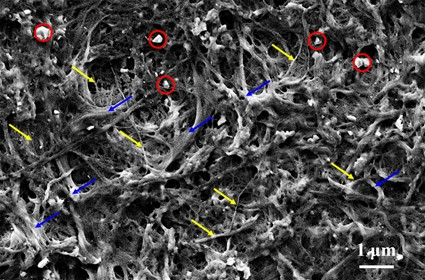Fig. 1. SEM image of sample No. 23; cellulose particles, CNFs, and large fibers are labeled by red circles, yellow arrows, and blue arrows, respectively

The diameter and distribution of CNFs and cellulose balls in the SEM images were analyzed using a previously published calculation method (Zhang et al. 2015b). All the responses (Fm) were thus collected and are listed in Table 4. The statistical analysis of these results after the orthogonal experiments was performed in two parts: SA and ANOVA.

Simple Analysis

The SA of the orthogonal experiments was used to find out the influence of a single factor on the CNF production by ball milling. The observations and conclusions by SA were on the three selected levels for each single factor.

The estimated marginal means of Factor A, i.e., mass ratio of milling ball to cellulose pulp, and its profile plot are shown in Table 5 and Fig. 2, respectively. The results disclose that the percentage of CNFs increased when the mass ratio of milling ball to cellulose pulp increased from 40% (level 1) to 60% (level 2) and finally to 80% (level 3). However, the slope of the profile plot in Fig. 2 decreased as the mass ratio increased. These experimental results could have been due to the fact that the higher mass ratio of milling ball to cellulose pulp provided a more direct contact surface area between the milling balls and fibers, which led to the larger effectiveness of CNF production. When the amount of zirconia milling balls reached a certain number, fibers were assumed to have full contact with the milling balls. This observation may have resulted in the insignificant increase in the yield of CNFs with increased mass ratio of milling ball to cellulose pulp.

Table 4. Results of Orthogonal Tests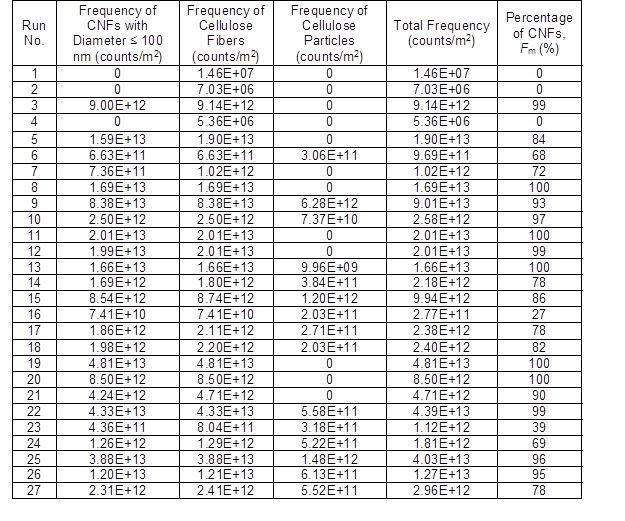Table 5. Estimated Marginal Means of Factor ARegarding Factor B (milling ball size), the estimated marginal means and its profile plot are shown in Table 6 and Fig. 3, respectively. The milling balls with diameters of 0.3 mm and 0.8 mm to 1.0 mm worked better than those with a diameter of 0.4 mm to 0.6 mm. This was likely due to the fact that the materials and densities of the different sized milling balls were different. The milling balls of 0.3 mm were made up of YDZ with a density of 1200 kg/m3, whereas the milling balls of 0.4 mm to 0.6 mm and 0.8 mm to 1.0 mm were composed of cerium-doped-zirconia (CDZ) with a density of 6200 kg/m3. For the same density, the milling balls of 0.8 mm to 1.0 mm produced more CNFs than those of 0.4 mm to 0.6 mm. For the milling balls with different densities, such experimental results were incomparable because the total contact surface area between milling balls and fibers during ball milling is dependent on both the size and the amount of milling balls, which is directly related to the density.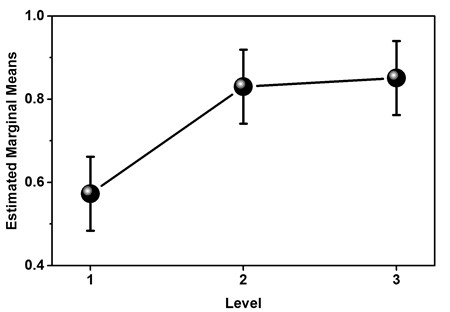Fig. 2. Profile plot for the factor analysis of mass ratio of milling ball to cellulose pulp

Table 6. Estimated Marginal Means of Factor B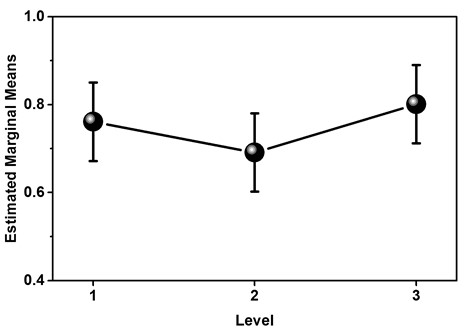Fig. 3. Profile plot for the factor analysis of milling ball diameter

When it comes to Factor C (milling time), the estimated marginal means and its profile plot are shown in Table 7 and Fig. 4, respectively. The experimental results indicated a proportional relationship between the milling time and the percentage of CNFs in the final suspension (Fig. 4), which also meant that the longer the milling time, the more CNFs that were obtained. This could have been attributed to the greater mechanical interactions between the milling balls and fibers in the suspension that was experienced during the longer milling time.

Table 7. Estimated Marginal Means of Factor C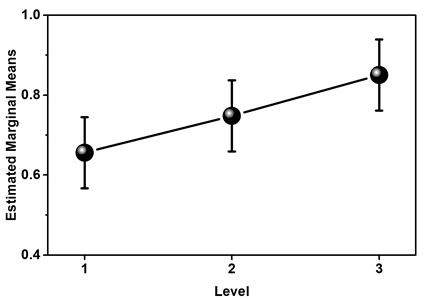Fig. 4. Profile plot for the factor analysis of milling time

The estimated marginal means of Factor D, i.e., concentration of sodium carbonate, and its profile plot are shown in Table 8 and Fig. 5, respectively. Interestingly, milling in the solution of 1 wt.% sodium carbonate (Level 2) had less of an influence on the percentage of CNFs than that without sodium carbonate (Level 1).

Table 8. Estimated Marginal Means of Factor DThe maximum number of CNFs was obtained in the solution of 2 wt.% sodium carbonate (Level 3). Such unexpected experimental results could have been related to sodium carbonate-induced hydrolysis of the cellulose during ball milling. Typically, hydrolysis of cellulose can break the cellulose and increase the number of cellulose particles (Fig. 1), thus decreasing the percentage of CNFs. As for the increased concentration of sodium carbonate, the cellulose particles were prone to dissolution, which increased the percentage of CNFs.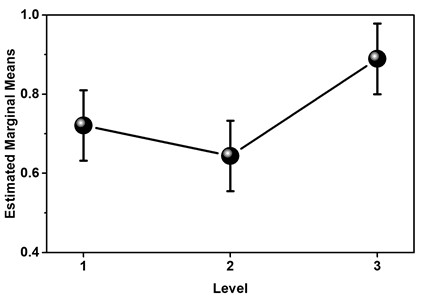Fig. 5. Profile plot for factor analysis of the concentration of sodium carbonate

ANOVA Analysis

The simple analysis presented above showed the effect of each single factor on the final production of CNFs.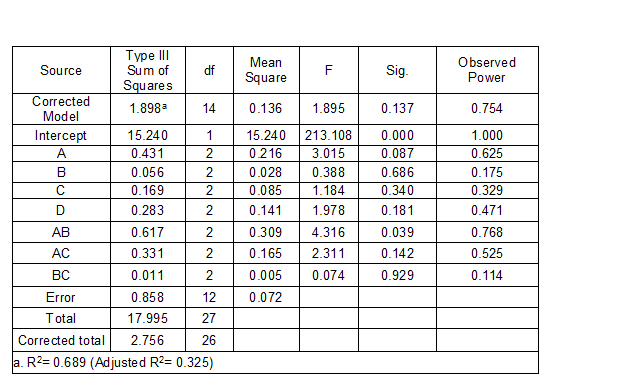Table 9. Tests of the Effects Caused by the Single Factors and the Interactions Between Factors

However, such an analysis is usually insufficient for the practical production, because it cannot consider the combined effects caused by the interactions between factors and realize all the optimal conditions of every factor at the same time. Therefore, it was necessary to distinguish the significant factors from the insignificant factors. In this study, an ANOVA calculation was performed to determine the relative influence of the single factors and the combined effects caused by the interactions between factors. The confidence levels were set between 90% and 95%, and the calculation results are listed in Tables 9 and 10.

Table 9 shows that all the mean squares of Factor B (0.028) and the interaction Factor BC (0.005) were much smaller than the error (0.072); this suggested that the effects caused by the single Factor B and interaction Factor BC were less significant than the error of the experimental system. The influences on the quality and yield of CNF caused by these two factors could be ignored; therefore, these two factors can be calculated as the error. Accordingly, the corrected sum of the square of the error includes the original error of the factor (0.858), the single Factor B (0.056), and the interaction Factor BC (0.011). Meanwhile, the corrected freedom includes the original freedom of the factor of error (12), the single Factor B (2), and the interaction Factor BC (2). All of the corrected values were calculated and are shown in Table 10.

Table 10. ANOVA Calculation Sheet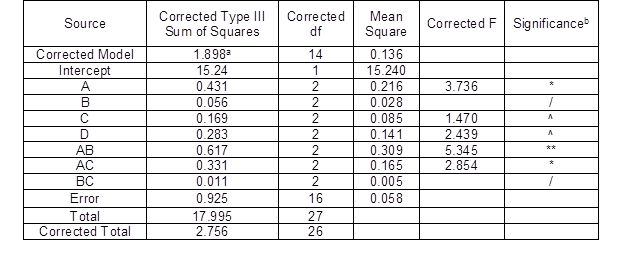Note: b. F0.9 (2, 16) = 2.67; F0.95 (2, 16) = 3.63 from F statistic table; / means the influence of the factor is insignificant and can be ignored; ^ means the influence of the factor is insignificant but cannot be ignored; * means the influence of the factor is significant; ** the influence of the factor is very significant

The judgment of significance is based on the F-value in Table 10. The F0.9 (2, 16) and F0.95 (2, 16) are the desired values in this test. If the F-value of the factor is larger than F0.95 (2, 16), the influence of this factor can be regarded as very significant. If the F-value is less than F0.95 (2, 16) while larger than F0.9 (2, 16), the influence of such factor is assumed to be less significant. If the F-value is less than F0.9 (2, 16), the influence of such factor is insignificant, but still cannot be ignored; whereas, if the factor is equal to an error, e.g., Factors B and BC, the influence of this factor should be considered so insignificant that it can be ignored.

Table 10 indicates that the significances of both the single and interaction factors were dramatically different. The influence of the mass ratio of milling ball to cellulose solid content (Factor A) was significant, while that of the milling ball size between 0.3 mm and 1.0 mm (Factor B) can be ignored; although the influences of milling time (Factor C) and concentration of sodium carbonate (Factor D) were insignificant, they could not be neglected. More importantly, the influences of interaction factors of mass ratio of milling ball to cellulose and milling ball size (Factor AB) and the mass ratio of milling ball to cellulose pulp and milling time (Factor AC) were very significant. In contrast, the influence of the interaction of milling ball size and milling time (Factor BC) was insignificant and could be ignored.

The above ANOVA analysis suggested the tendency of the influences of factors on the production of CNFs by ball milling from softwood pulp. The most important single factor was the mass ratio of milling ball to cellulose solid content, which can determine the influence of milling ball size and milling time. In addition, the interactions between the mass ratio of milling ball to cellulose pulp and other single factors showed very significant influences on the yield of CNFs. The introduction of alkali to the system had a less significant influence on the yield of CNFs, while it could increase the number of steps in production. Therefore, it is not recommended for practical production. These findings are meaningful for the mass production of CNFs using ball milling.

Mini Scale-up Production of CNF by Ball Milling

Based on the findings from the ANOVA, the described ball milling technique could be scaled-up easily by controlling the mass ratio of milling ball to cellulose pulp (R). The R was estimated theoretically to provide an approximate idea for the data setting during production.

If the CNF is assumed as a cylindrical fibre with a diameter of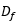and a length of L, its aspect ratio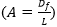can be roughly regarded as 100. Therefore, the surface area of the CNF (Sf) can be calculated by: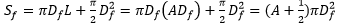(1)

Because ≈ 100 ≫ 1/2, the surface area of the CNF (Sf) can be roughly written as: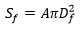(2)

The surface area of each spherical milling ball (Sb) can be calculated by,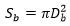(3)

where Db is the diameter of the spherical milling ball (mm).

Theoretically, if the milling balls and fibers are fully contacted during ball milling, the mass ratio of milling ball to cellulose pulp can be obtained by the calculation,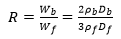(4)

where R is the mass ratio of milling ball to cellulose pulp, Wb and Wfare the masses of milling balls and fibers, respectively;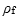is the density of CNFs, which is approximately 1500 kg/cm3, and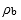is the density of milling balls. Theof milling balls with a diameter of 0.3 mm was 1184 kg/cm3, while that of milling ball diameters of 0.4 mm to 0.6 mm and 0.8 mm to 1.0 mm was 6200 kg/cm3. In a practical situation, thevaries during ball milling, as it usually decreases from the micro- to nano-scale. Therefore, the ratio of milling ball to cellulose pulp (R) could be designed by the desirable CNF size before producing according to Eq. 4. In this study, the diameters of the milling balls and raw pulp were around 0.3 mm and 40 um, respectively, whereas, the target CNF diameter was approximately 100 nm. Accordingly, in theory the optimal R should be designed in the range of 4 to 1579; otherwise, it is difficult to ensure the yield of CNFs.

CONCLUSIONS

1. The preparation of CNFs by ball milling from softwood pulp was systematically studied by multiple factor analysis using the Taguchi method and ANOVA analysis.
2. Among the major single factors possibly influencing the production of CNF, milling ball size was a factor that can be neglected, while mass ratio of milling ball to cellulose pulp was the most significant factor. In addition, the interactions between the mass ratio of milling ball to cellulose pulp and other single factors showed very significant influence on the yield of CNF.
3. The approximation of the mass ratio of milling ball to cellulose pulp has been proposed for the production of CNF with different target diameters. The results demonstrated this to be effective for the mini scale-up production of CNF using ball milling. This calculation method proved the possibility of the application of ball milling technique on the mass production of CNF from sustainable resources without using any hazardous organic solvent and provides meaningful and effective guidance for data selection during mass production.

ACKNOWLEDGMENTS

Liyuan Zhang and Wei Shen acknowledge the Australian Research Council Discovery Project (DP1094179 and 140100052) and the Zhejiang International Science and Technology Cooperation Project (2015C34014). Xungai Wang would like to acknowledge support from the Australian Research Council World Class Future Fibre Industry Transformation Research Hub (IH140100018). Juliang Yin thanks the Natural Science Foundation of China (61573006). Hui He and Ruoyang Chen thank Monash Graduate Education and the Faculty of Engineering for scholarships. Liyuan Zhang also thank IDP Mobility Scholarship (2008-2012) for the support.

REFERENCES CITED

Abdi, H., Williams, L. J., and Valentin, D. (2013). “Multiple factor analysis: Principal component analysis for multitable and multiblock data sets,” WIREs Computational Statistics 5(2), 149-179. DOI: 10.1002/wics.1246

Abdul Khalil, H. P. S., Bhat, A. H., and Ireana Yusra, A. F. (2012). “Green composites from sustainable cellulose nanofibrils: A review,” Carbohydr. Polym. 87(2), 963-979. DOI: 10.1016/j.carbpol.2011.08.078

Abitbol, T., Rivkin, A., Cao, Y., Nevo, Y., Abraham, E., Ben-Shalom, T., Lapidot, S., and Shoseyov, O. (2016). “Nanocellulose, a tiny fiber with huge applications,” Curr. Opin. Biotech. 39, 76-88. DOI: 10.1016/j.copbio.2016.01.002

Bodin, A., Backdahl, H., Risberg, B., and Gatenholm, P. (2007). “Nano cellulose as a scaffold for tissue engineered blood vessels,” Tissue Eng. 13(4), 885.

Boluk, Y., Lahiji, R., Zhao, L., and McDermott, M. T. (2011). “Suspension viscosities and shape parameter of cellulose nanocrystals (CNC),” Colloid. Surface. A. 377(1), 297-303. DOI: 10.1016/j.colsurfa.2011.01.003

Brown, A. J. (1886). “On an acetic ferment which forms cellulose,” J. Chem. Soc. Trans. 49, 432-439. DOI: 10.1039/CT8864900432

Brown, M. R., Czaja, W., Jeschke, M., and Young, D. J. (2006). “Multiribbon nanocellulose as a matrix for wound healing,” U.S. Patent No. 8951551 B2.

Chen, R. Y., Zhang, L. Y., Li, X., Ong, L., Soe, Y. G., Sinsua, N., Gras, S. L., Tabor, R. F., Wang, X. G., and Shen, W. (2017). “Trace analysis and chemical identification on cellulose nanofibers-textured SERS substrates using the “coffee ring” effect,” ACS Sens. 2(7), 1060-1067. DOI: 10.1021/acssensors.7b00403

Chen, W., Yu, H., Liu, Y., Hai, Y., Zhang, M., and Chen, P. (2011). “Isolation and characterization of cellulose nanofibers from four plant cellulose fibers using a chemical-ultrasonic process,” Cellulose 18(2), 433-442. DOI: 10.1007/s10570-011-9497-z

Czaja, W., Krystynowicz, A., Bielecki, S., and Brown, J. R. M. (2006). “Microbial cellulose – the natural power to heal wounds,” Biomaterials 27(2), 145-151. DOI: 10.1016/j.biomaterials.2005.07.035

Kim, C. W., Kim, D. S., Kang, S. Y., Marquez, M., and Joo, Y. L. (2006). “Structural studies of electrospun cellulose nanofibers,” Polym. J. 47(14), 5097-5107. DOI: 10.1016/j.polymer.2006.05.033

Kuznetsova, A., Christensen, R. H. B., Bavay, C., and Brockhoff, P. B. (2015). “Automated mixed ANOVA modeling of sensory and consumer data,” Food Qual. Prefer. 40, 31-38. DOI: 10.1016/j.foodqual.2014.08.004

Lavoine, N., Desloges, I., Dufresne, A., and Bras, J. (2012). “Microfibrillated cellulose – its barrier properties and applications in cellulosic materials: A review,” Carbohydr. Polym. 90(2), 735-764. DOI: 10.1016/j.carbpol.2012.05.026

Nogi, M., Kim, C., Sugahara, T., Inui, T., Takahashi, T., and Suganuma, K. (2013). “High thermal stability of optical transparency in cellulose nanofiber paper,” Appl. Phys. Lett. 102(18), 181911. DOI: 10.1063/1.4804361

Rama Rao, S., and Padmanabhan, G. (2012). “Application of Taguchi methods and ANOVA in optimization of process parameters for metal removal rate in electrochemical machining of Al/5%SiC composites,” Int. J. Eng. Res. Appl. 2(3), 192-197.

Salas, C., Nypelö, T., Rodriguez-Abreu, C., Carrillo, C., and Rojas, O. J. (2014). “Nanocellulose properties and applications in colloids and interfaces,” Curr. Opin. Colloid In. 19(5), 383-396. DOI: 10.1016/j.cocis.2014.10.003

Siró, I., and Plackett, D. (2010). “Microfibrillated cellulose and new nanocomposite materials: A review,” Cellulose 17(3), 459-494. DOI: 10.1007/s10570-010-9405-y

Wertz, J., and Schneiders, I. (2009). “Filtration media: Advantages of nanofibre coating technology,” Filtr. Separat. 46(4), 18-20. DOI: 10.1016/S0015-1882(09)70156-9

Wicklein, B., Kocjan, A., Salazar-Alvarez, G., Carosio, F., Camino, G., Antonietti, M., and Bergström, L. (2015). “Thermally insulating and fire-retardant lightweight anisotropic foams based on nanocellulose and graphene oxide,” Nat. Nanotechnol. 10(3), 277-283. DOI: 10.1038/nnano.2014.248

Yang, W. H., and Tarng, Y. S. (1998). “Design optimization of cutting parameters for turning operations based on the Taguchi method,” J. Mater. Process. Tech. 84(1-3), 122-129. DOI: S0924-0136(98)00079-X

Zhang, L.Y., Batchelor, W., Varanasi, S., Tsuzuki, T., and Wang, X. G. (2012). “Effect of cellulose nanofiber dimensions on sheet forming through filtration,” Cellulose, 19(2), 561-574. DOI: 10.1007/s10570-011-9641-9

Zhang, L. Y., Li, X., Ong, L., Tabor, R. F., Bowen, B. A., Fernando, A. I., Nilghaz, A., Garnier, G., Gras, S. L., Wang, X. G., and Shen, W. (2015a). “Cellulose nanofibre textured SERS substrate,” Colloid. Surface. A. 468, 309-314. DOI: 10.1016/j.colsurfa.2014.12.056

Zhang, L. Y., Tsuzuki, T., and Wang, X. G. (2010). “Preparation and characterization on cellulose nanofiber film,” Mater. Sci. Forum 654-656, 1760-1763. DOI: 10.4028/www.scientific.net/MSF.654-656.1760

Zhang, L. Y., Tsuzuki, T., and Wang, X. G. (2015b). “Preparation of cellulose nanofiber from softwood pulp by ball milling,” Cellulose22(3), 1729-1741. DOI: 10.1007/s10570-015-0582-6

Zirehpour, A., Rahimpour, A., Jahanshahi, M., and Peyravi, M. (2014). “Mixed matrix membrane application for olive oil wastewater treatment: Process optimization based on Taguchi design method,” J. Environ. Manage. 132, 113-120. DOI: 10.1016/j.jenvman.2013.10.028

Article submitted: October 6, 2017; Peer review completed: December 16, 2017; Revised version received: January 31, 2018; Accepted: February 1, 2018; Published: February 9, 2018.

DOI: 10.15376/biores.13.2.2397-2410